• 2020-09-28 09:27:04

其实很简单，只需要在原来基础上多加一个星号就可以了。

表格横跨两栏代码

\begin{table} … \end{table}

图形横跨两栏代码

\begin{figure*} … \end{figure*}

更多相关内容
• 文章目录 1、单双栏排版 2、双倍行距 3、图片表格跟随对应的段落，而非置页顶 ...如果删掉onecolumn即双栏排版。 2、双倍行距 添加包 \usepackage{setspace} 之后在需要调节行距的段落前输入： \doub

## 1.单双栏排版（以IEEE模板为例）

单栏：\documentclass[journal, onecolumn]{IEEEtran}
双栏：\documentclass[journal, twocolumn]{IEEEtran}

如果删掉onecolumn即双栏排版。

## 2.双倍行距

添加包 \usepackage{setspace}

之后在需要调节行距的段落前输入：

\doublespacing
\singlespacing
\onehalfspacing


三选一即可，我是在\begin{document}前输入的\doublespacing

## 3.图片表格跟随对应的段落，而非置页顶

首先在编辑器头部加入宏包 \usepackage{float}，然后在插入图片的语句后加个[H]即可，如：

\begin{figure}[H]
\centering
\includegraphics[width=\linewidth]{XXXX.pdf}
\caption{XXXXXX}
\label{XXXXXX}
\end{figure}


figure or table都适用。

## 4.显示行号

\usepackage{lineno} % 这个包就是latex自带的添加行号的包了

\linenumbers        % 全文从头到尾编号

\pagewiselinenumbers   %按页重新编号

\begin{document}	% 文章开始


## 5.调节abstract与标题的距离

\maketitle
\vspace{-1.6cm}  %1.6cm即数值，自行调节即可。

\begin{abstract}

展开全文• Latex 双栏模式下表格太长怎么办？这一条够简单！”点击Latex 双栏模式下表格太长怎么办？作　者：marsggbo出　处：https://www.cnblogs.com/marsggbo科技文档排版时，有时表格可能一页都装不下，或者双栏模板单栏...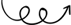Latex 双栏模式下表格太长怎么办？这一条够简单！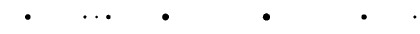点击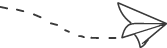# Latex 双栏模式下表格太长怎么办？

作　　者：marsggbo出　　处：https://www.cnblogs.com/marsggbo

科技文档排版时，有时表格可能一页都装不下，或者双栏模板单栏不够长，如果用原始的table包的话很可能就会溢出了，所以很自然的一个想法是让表格自动换页、竖排等，网上很多资料都是推荐longtable，但在双栏文章模板里面用这个包可能会有问题，比如table只是会浮在文字的上面、caption的显示也常常有问题，试了很久终于找到了解决办法，而且很简单，只需要缩放一下table就好，方法如下：

\begin{table*}[!ht]\scalebox{0.7}{  \begin{tabular}    ...  \end{tabular}}\caption{}\end{table*}

参考: https://stackoverflow.com/questions/2563498/making-latex-tables-smaller

参考: https://www.cnblogs.com/marsggbo/p/13179135.html

作者：marsggbo

出处：https://www.cnblogs.com/marsggbo喜欢就点个 在看 吧！展开全文• 最近在写论文，整体是双栏排版 需要在单栏插入两个图，并列显示 在网上搜了一圈，主流解决方案如下，参考自知乎 \begin{figure}[htbp] \centering \begin{minipage}[t]{0.48\textwidth} \centering \...

# 问题

最近在写论文，整体是双栏排版
需要在单栏插入两个图，并列显示
在网上搜了一圈，主流解决方案如下，参考自知乎

\begin{figure}[htbp]
\centering
\begin{minipage}[t]{0.48\textwidth}
\centering
\includegraphics[width=5cm]{lsun_ptxt.png} %修改图片名称
\caption{1} % 添加图片描述
\end{minipage}
\begin{minipage}[t]{0.48\textwidth}
\centering
\includegraphics[width=5cm]{lsun_ctxt.png}
\caption{2}
\end{minipage}
\end{figure}


但是最后的效果变成了，单栏双图垂直显示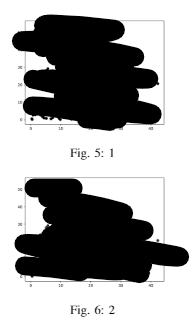# 解答

我们可以在一个单独figure中，插入两个minipage

\begin{figure}[htbp]
\begin{minipage}[t]{0.45\linewidth}
\includegraphics[width=\linewidth]{figs1.eps} # 修改图片名称
\caption{caption1} # 修改描述
\label{f1}
\end{minipage}%
\hfill%
\begin{minipage}[t]{0.45\linewidth}
\includegraphics[width=\linewidth]{figs2.eps}
\caption{caption2}
\label{f2}
\end{minipage}
\end{figure}


效果如下图，完美解决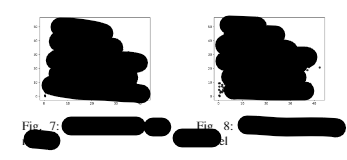参考自这里

展开全文• Latex文档插图，西北农林科技大学耿楠老师Latex排版上课笔记
• Elsevier 双栏模板下的长公式 \documentclass[5p,preprint,12pt]{elsarticle} \usepackage{amsmath} \usepackage{...（1）LaTeX双栏模板插入通栏公式（跨栏插图） （2）cuted – Mixing onecolumn and twocolumn modes
• 因此找到了一种更简单的方法：在table or figure里多加一个星号就实现了完美的单栏表格或图片排版双栏的文章里。 表格横跨两栏代码 \begin{table*} … \end{table*} 图形横跨两栏代码 \begin{figure*} … \end{...
• 在table or figure里多加一个星号就实现了完美的单栏表格或图片排版双栏的文章里。 表格横跨两栏代码 \begin{table*} … \end{table*} 图形横跨两栏代码 \begin{figure*} … \end{figure*}
• Latex栏排版中图片、表格需要设置为一栏显示，在{figure}、{table}后加上 * \begin{figure*} …… \end{figure*} 实例如下： \begin{figure*}[!t] \centering \includegraphics[width=6in]{fig1.png} \label{...
• 1. 照片双栏格式插入 \begin{figure} \includegraphics[width=\linewidth]{qian.jpg} \caption{Example of a figure caption.} \label{qian.jpg} \end{figure} 2. 照片单栏格式插入 \begin{figure*} \...ieee论文
• latex双栏模板中使用 通栏的公式或者图 通栏的图 在原来插图的环境中的 figure后加*；具体如下 \begin{figure*}[ht] \label{fig1} \centering \includegraphics[width = 3in]{Fig1.pdf} \caption{The ...
• LaTeX文档的最开始声明使用单栏或双栏可选项： 单栏 \documentclass[onecolumn]{article} 双栏 \documentclass[twocolumn]{article}macos
• ## Latex 表格双栏 单栏

千次阅读 2020-08-14 10:52:10
双栏 \begin{table*} ... \end{table*} 单栏 \begin{table} ... \end{table}
• https://blog.csdn.net/archielau/article/details/7958173 https://blog.csdn.net/jorg_zhao/article/details/80703981
• 在导言区加入如下宏包即可！不需要其他任何操作！ \usepackage{flushend}
• \begin{figure}[htbp] %注意，这里设置是关键 \centering \includegraphics[width=\linewidth,scale=1.00]{tight.png} %[]里面的参数自己可根据需要调整...这是两只占一 如果要横框两，figure后加* ...
• LaTeX中最实用的双栏转单栏公式表格插入插入公式插入表格 插入公式 \begin{figure*}[b] {\noindent} \rule[-10pt]{18.07cm}{0.1em} \begin{equation} e^{i\pi}+1=0 \end{equation} \end{figure*} ...
• LaTeX文档的最开始使用单栏或双栏可选项： 单栏： \documentclass[onecolumn]{article} 双栏： \documentclass[twocolumn]{article} 对应于IEEE的模板可以修改为 \documentclass[journal,onecolumn]{IEEEtran}
• （3）longtable在双栏或者多栏中使用, 加*号。 \begin{longtable*} ... \end{longtable*} % 第一页表头内容 \endfirsthead % 跨页表头内容 \endhead % 跨页表尾内容 \endfoot % 最后一页表尾内容 \endlastfoot ...
• \usepackage{wrapfig} \begin{wrapfigure}{l}[0em]{0.15\textwidth}%靠文字内容的左侧 \includegraphics[width=0.15\textwidth]{figure/XXX.png} \end{wrapfigure} \textbf {XXX} received the B.S....
• 直接使用　\usepackage{flushend}　即可
• 方法1: IEEE官方修改（本文推荐） \begin{figure*} \begin{equation} \label{eq-13} +++++++++++++++++ Put equation here +++++++++++++++++ \end{equation} \hrulefill % \vspace*{4pt} ...
• 1.问题：怎么在双栏排版中，让占据两栏的表格出现在页面顶端？ 2.解决： 1）图片：（占据两栏显示在页面顶端） 2）表格：（占据两栏显示在页面顶端） 1.问题： 怎么在双栏排版中，让通栏的表格出现在页面顶端...
• latex双栏排版中的图表及公式通栏 \begin{multicols}{2} Bla bla... \end{multicols} % \leftsep \begin{equation} \label{eqn:planar:Hz02} \left \{\begin{array}{l} \delta A + \...
• 在导言区，加入stfloats包：\usepackage{stfloats}接着将htbp改为hb：\section{Introduction}\begin{figure*}[hb]\centering\includegraphics[scale=0.4]{xxx.eps}\caption{xxxxxxxxxx}\label{fig_framework}\end{...
• 关于的入门，可以参考：《LaTeX环境配置》，和《LaTeX入门(一)》当然，我不打算继续从 入门(二)开始写起，关于这个新的专题：学习指南，将会一直写下去，与 R 语言系列一样不断地更新，同时，我希望，尽可能地解释...
• 只需\begin{table*}[]就可以实现...[p] 这个是将图片设置为浮动状态，也就是可以根据系统排版的，自动放置图片的位置 [htb] 优先放置在最佳位置，然后将其放在顶端最后放在底部。我们知道一般对于论文的输入可不是几...